• 【matlab代码】动态规划求解tsp问题
2021-04-03 09:57:50

最近在做毕业设计，从0学习了matlab和一些启发式算法，其中有个部分为tsp问题，思来想去想复现参考论文中的动态规划求解，网上看了一些资料，代码都是c++或者java的，这里分享下自己用matlab写的代码：
注：参考资料为https://blog.csdn.net/hytfly/article/details/95739609
本算法相关问题情景和解题思路均通过学习参考资料所获，欢迎各位在评论区交流学习matlab 代码规范
更多相关内容
• 该文档使用Java语言编写了一个通用的TSP问题求解方法，不仅进行了代码求解，还根据实际例子进行了手动求解和介绍，适合旅行商入门，以及Java语言的学习，附带源码和伪代码，以及详细的解释。
• 程序入口，求解TSP问题并可视化 cities.json：json数据 [[88, 88], [93, 13], [17, 20], [13, 70]] tool.py：工具模块 # -*- coding:utf-8 -*- import math import json import random ...

设计思路：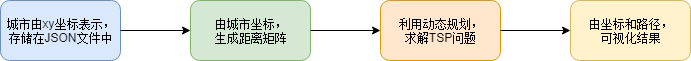程序清单：

 文件名 定位 作用 cities.json json数据 存储城市坐标信息 tool.py 工具模块 提供读取JSON数据、生成随机点、生成距离矩阵等函数 main.py 主模块 程序入口，求解TSP问题并可视化

cities.json：json数据

[[88, 88], [93, 13], [17, 20], [13, 70]]

tool.py：工具模块

# -*- coding:utf-8 -*-

import math

import json

import random

# 生成一定范围的随机数

def random_double(_min, _max):

return random.random() * (_max - _min) + _min

# 生成随机城市坐标

def random_cities(_min, _max, num):

return [[random_double(_min, _max), random_double(_min, _max)] for _ in range(num)]

# 计算欧式距离

def distance(c1, c2):

return math.sqrt(square_distance(c1, c2))

# 计算平方欧式距离

def square_distance(c1, c2):

return (c1 - c2) ** 2 + (c1 - c2) ** 2

# 由城市坐标列表，生成距离矩阵

def distance_matrix(_cities):

length = len(_cities)

matrix = [[-1.0] * length for _ in range(length)]

for i in range(length - 1):

for j in range(i + 1, length):

matrix[i][j] = matrix[j][i] = distance(_cities[i], _cities[j])

return matrix

# 从json文件中，读取城市信息

json_path = 'cities.json'

with open(json_path, 'r') as f:

return json.load(f)

main.py：主模块

# -*- coding:utf-8 -*-

import matplotlib.pyplot as plt

class Solution:

def __init__(self, _matrix, start_node):

# 距离矩阵

self.matrix = _matrix

# 节点数目

self.height = len(self.matrix)

# 宽度

self.width = 2 ** self.height

# 开始节点

self.start_node = start_node

# 记录处于x节点，未经历M个节点时

# 矩阵储存x的下一步是M中哪个节点

self.array = [ * self.width for _ in range(self.height)]

def tsp(self):

# 开始节点

s = self.start_node

# 节点数目

num = self.height

# 未遍历节点集合

_cities = list(range(num))

_cities.pop(s)

# 求解函数

return self.solve(s, _cities)

@staticmethod

def transfer(sets):

_sum = 0

for s in sets:

_sum += 2 ** s  # 二进制转换

return _sum

def solve(self, node, future_sets):

# 迭代终止条件，

# 表示没有了未遍历节点，直接连接当前节点和起点即可

if len(future_sets) == 0:

return self.matrix[node][self.start_node]

# node如果经过future_sets中节点，最后回到原点的距离

distance = []

# 遍历未经历的节点

for i in range(len(future_sets)):

s_i = future_sets[i]

# 访问s_i，更新当前未访问节点集合

copy = future_sets[:]

copy.pop(i)

# 递归,动态规划递推方程

distance.append(self.matrix[node][s_i] + self.solve(s_i, copy))

# 最小距离

d = min(distance)

# node需要连接的下一个节点

next_one = future_sets[distance.index(d)]

# 未遍历节点集合

c = self.transfer(future_sets)

# (当前节点，未遍历节点集合)-->下一个节点

self.array[node][c] = next_one

return d

if __name__ == '__main__':

# 从JSON读取数据

# 生成距离矩阵

mat = distance_matrix(cities)

# 调用tsp

S = Solution(mat, 0)

S.tsp()

# 开始回溯

lists = list(range(len(S.matrix)))

start_node = S.start_node

# 生成路径

output_path = list()

while len(lists) > 0:

lists.pop(lists.index(start_node))

# 未访问节点集合 矩阵中的y坐标

y = S.transfer(lists)

# 下一节点

next_node = S.array[start_node][y]

# 添加下一组合

output_path.append([start_node, next_node])

# 从下一节点出发

start_node = next_node

# 输出城市列表

print('城市坐标集合为：')

for city in cities:

print(city, end=' ')

print()

# 距离矩阵

print('距离矩阵为：')

for row in mat:

for col in row:

print('%10.2f' % col, end='   ')

print()

# 输出路径

print('最优路径为：')

for i in output_path:

print(i, '-->', end='')

print('0')

# 画散点图

i = 0

for city in cities:

plt.scatter(city, city)

plt.annotate("City%d(%d, %d)" % (i, city, city), (city + 2, city + 2))

i += 1

# 画出路径

for start_node, next_node in output_path:

cs = cities[start_node]

cn = cities[next_node]

plt.plot([cs, cn], [cs, cn])

# 不显示坐标轴

plt.axis('off')

# 绘图

plt.show()



TSP问题

输出结果：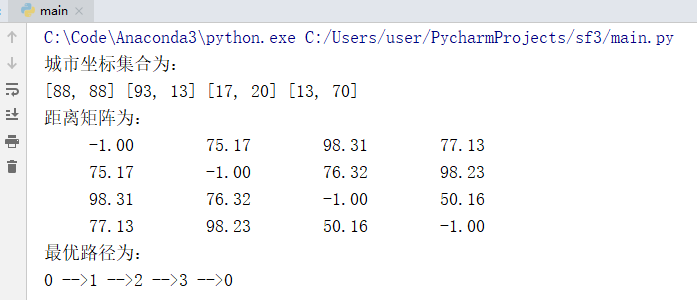绘图结果：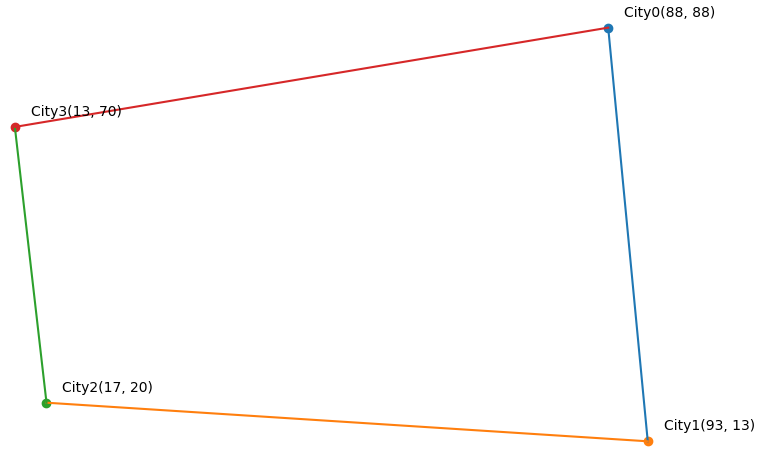展开全文• 使用动态规划方法求解TSP问题 这两天看到了一个用动态规划方法求解TSP问题的案例，原文代码是用C++写的，本人照着写成了java的代码，可以运行出相同的最后结果，但是不知道该如何得到最终的访问城市序列。 但是...

# 使用动态规划方法求解TSP问题

这两天看到了一个用动态规划方法求解TSP问题的案例，原文代码是用C++写的，本人照着写成了java的代码，可以运行出相同的最后结果，但是不知道该如何得到最终的访问城市序列。

但是其中的每个步骤已经弄得很详细了，算是把明白的记录下来，不懂得留下来有机会再研究。

感谢原作者，感谢感谢。

这个就百度就好了

# 2. 将DP应用到TSP问题中，建立动态规划模型。

具体解TSP问题时，没有必要将所有的DP步骤全部写出来。将状态转移方程写明白就可以理解代码了。下面仔细讲解状态转移方程。

### 2.1 指标函数 d ( i , V' )

i 表示当前路径中最后一个访问的城市节点（将要去的下一个城市，决策变量）；V' 表示已经访问过的城市集合（状态）。

d ( i , V' ) 表示从初始点出发，经过V'集合中的所有城市一次，最终到达城市 i，的最小花费。

假设s为起点，则d ( t , { s, q, t } ) = 0 表示：s -> q -> t 这条路径的最小花费

### 2.2 状态转移方程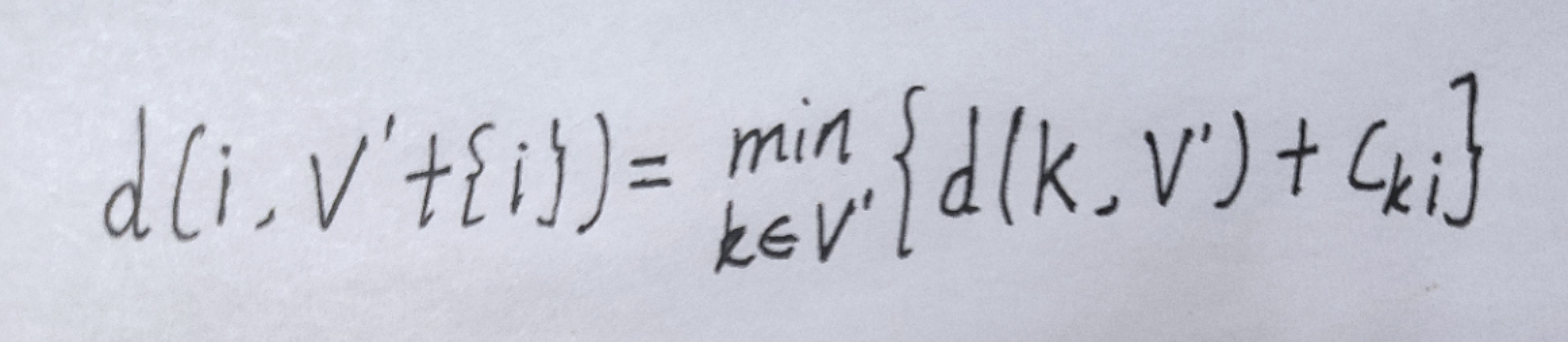d(i, V' + { i }) 表示的是：s -> V' -> k -> i ，这条路径的最小花费。

要想找到下一个访问城市i，以达到最小花费，需要在V'（已访问城市集合）中找到路径末端的城市，用路径末端城市与下一个可能的访问城市进行匹配，找到最小花费的匹配。

# 3. 状态压缩（这里仅介绍本文用到的状态压缩方式）

所谓状态压缩，就是利用二进制以及位运算来实现对于本来应该很大的数组的操作。而求解动态规划问题，很重要的一环就是状态的表示，一般来说，一个数组即可保存状态。但是有这样的一些题目，它们具有DP问题的特性，但是状态中所包含的信息过多，如果要用数组来保存状态的话需要四维以上的数组。于是，我们就需要通过状态压缩来保存状态，而使用状态压缩来保存状态的DP就叫做状态压缩DP。（以上是废话）

假设问题中一共有四个访问点，编号为：0，1，2，3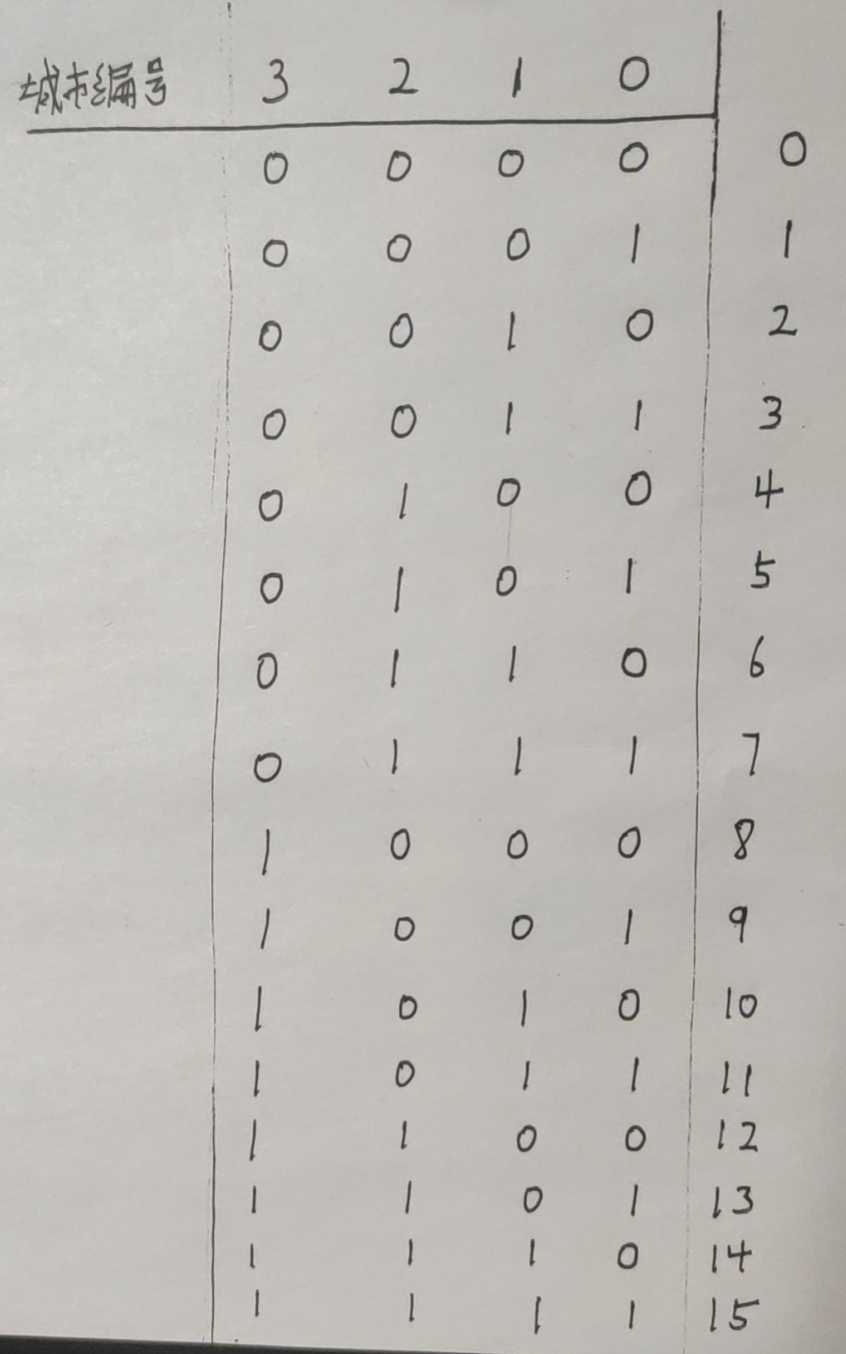在上图中，最右遍那一列，0 ~ 15即为V'的值，用十进制数字来保存当前状态。

例如：当V' = 3时，3的二进制为左边的四列 0 0 1 1 ，表示已经访问过的城市集合中有城市0城市1

而且，通过左移运算（<<），计算出n个城市节点一共有多少种可能的状态。例如：四个城市节点时，(1 << 4) = 16，一共有16种状态。

# 4. 代码部分

代码部分是我照着那个朋友的C++代码直接写的java代码，可能会有还未发现的错误。希望朋友们指正。就其中三点做主要说明。

### 4.1 过滤1

这句代码在sovle()函数中， j 为下一步要访问的城市编号，i（状态）为已经访问过的城市集合，当状态 i 中已经包含了城市 j ，则过滤掉这个状态，跳出本次循环。

 if ((i & (1 << j)) != 0) continue;

例如：i = 12 , j = 4 。将 i j 都转化为二进制：i = 1 1 0 0 , j = 0 1 0 0 。通过位运算&，可以判断出 i & j = 0 1 0 0 ，此时在状态 i 中已经访问过了城市 j 。

此种情况下，就可以跳过i = 12 的状态，遍历下一个状态。

### 4.2 过滤2

这句代码在sovle()函数中，i（状态）为已经访问过的城市集合，用来过滤掉不是从城市0出发的状态。

if ((i & 1) == 0) continue;

例如：i = 12 ， i 转化为二进制：i = 1 1 0 0 。将1转化为二进制：1 = 0 0 0 1，这表示路径中只有出发的城市0。通过位运算&，可以判断出 i & j = 0 0 0 0 ，此时在状态 i 不是从城市0出发

### 4.3 在状态i中寻找

这句代码在sovle()函数，据说有两个功能：确保 k 节点已经在集合 i 中、确保 k 是上一步转过来的。

“ 确保 k 节点已经在集合 i 中” ，这个功能的原理在4.1中已经有讲解。

但是 “确保 k 是上一步转过来的 ” 这个功能还没发现是如何实现的。

只有实现了这个功能，才能保证将城市 j 连接到了路径的末端，而不是连接到了路径中的某个城市。

if (((i & (1 << k)) > 0))

这个还有待解决

# 5 代码部分

package TSP01;

import java.io.File;
import java.io.FileNotFoundException;
import java.util.*;

public class Main {
final static double INF = 999999;
static int N; // 城市数量
static double distance[][]; // 距离矩阵
static Vertex vertexs[]; // 储存城市结点的数组
static double dp[][]; // 存储状态
// dp[i][j]：j表示状态，i表示当前城市
static int p[]; // p[i]的值表示i节点的前驱节点
static double ans = INF; // 总距离
static int pre[]; // 索引为状态，值为此状态中上一次加入到状态数组中的车辆

public static void main(String[] args) throws FileNotFoundException {
// 1.读取数据
input("src\\TSP01\\a.txt", 1);
// 2.初始化状态
init_dp();
// 3.解问题
solve();
// 4.输出答案
//  4.1 按顺序将逆序访问序列添加到list链表中
int t = 0;
while(p[t]!=0){
t = p[t];
}
//  4.2 反转列表
Collections.reverse(list);
//  4.3 输出正序的城市访问序列
for (int i = 0; i < list.size(); i++) {
System.out.print(list.get(i) + " -> ");
}
System.out.println();
System.out.println("总路程为：" + ans);
for (int i = 0; i < N; i++) {
System.out.println(i + "的前置节点为：" + p[i]);
}
}

/**
* 读取数据（数据类型有两种，1是矩阵，0是二位数组）
*
* @param filename 文件名
* @param type     数据类型
*/
public static void input(String filename, int type) throws FileNotFoundException {
File file = new File(filename);
Scanner sc = new Scanner(file);
if (type == 1) {
N = sc.nextInt(); // 城市数量
p = new int[N];
distance = new double[N][N];
for (int i = 0; i < N; i++) {
for (int j = 0; j < N; j++) {
distance[i][j] = sc.nextDouble();
}
}
} else if (type == 0) {
N = sc.nextInt(); // 城市数量
p = new int[N];
vertexs = new Vertex[N];
distance = new double[N][N];
while (sc.hasNext()) {
int i = sc.nextInt() - 1; // 城市编号（从0开始）
vertexs[i] = new Vertex();
vertexs[i].id = i + 1;
vertexs[i].x = sc.nextDouble();
vertexs[i].y = sc.nextDouble();
}
for (int i = 0; i < N; i++) {
distance[i][i] = 0;
for (int j = 0; j < i; j++) {
distance[i][j] = edc_2d(vertexs[i], vertexs[j]);
distance[j][i] = distance[i][j];
}
}
} else {
System.out.println("类型输入错误");
}
sc.close();
}

// 计算两点之间的距离
public static double edc_2d(Vertex a, Vertex b) {
return Math.sqrt((a.x - b.x) * (a.x - b.x) + (a.y - b.y) * (a.y - b.y));
}

// 初始化状态
public static void init_dp() {
dp = new double[(1 << N) + 5][]; // 富余出5个
for (int i = 0; i < (1 << N); i++) { // 遍历所有状态
dp[i] = new double[N + 5];
for (int j = 0; j < N; j++) { // 每个状态下出发点到达每个点的距离
dp[i][j] = INF;
}
}
}

// 解决问题
public static void solve() {
int M = (1 << N); // 状态的个数
pre = new int[M];
pre = 0; // 初始化为0
dp = 0; // 确定出发点
// 下面的每个十进制的i转化成N位的二进制，就可以代表当前的状态。0代表没有经过这个点，1代表已经过这个点。
for (int i = 1; i < M; i++) { // 遍历每种状态
for (int j = 1; j < N; j++) { // 每种状态下遍历每个可访问的下一个城市
// 过滤1（按位与运算）：过滤掉状态中已经包含了城市节点j的状态
if ((i & (1 << j)) != 0) continue;

// 过滤2（按位与运算）：默认必须从0号城市开始出发，过滤掉不是从0号城市出发的状态
if ((i & 1) == 0) continue;

// 尝试使用V'集合中的节点连接下一个节点。...->k->j
for (int k = 0; k < N; k++) {
// 1. 确保k节点已经在集合中
// 2. 确保k是上一步转过来的（如何确保源代码中也没有做到）
// 只有当k是上一步转过来的，才可以得到一个TSP环，而不是其中有多个子环
// 这个问题在源代码中也没有得到解决
if (((i & (1 << k)) > 0)) {
// dp[(1<<j)][j] 表示的是当j点即为出发点时的情况
// dp[(1<<j)][j]中，(1<<j)表示的状态是计算不到这一步的，因此此种状态表示以j为出发点，不符合上面要求
if (dp[(1 << j) | i][j] > dp[i][k] + distance[k][j]) {
dp[(1 << j) | i][j] = dp[i][k] + distance[k][j]; // 状态转移方程

pre[i] = j;
String q = Integer.toBinaryString(i);
System.out.println("i= " + i + " : " + q + "  p[" + j + "] = " + k);
}
if(dp[i][j] > dp[i][k] + distance[k][j]){
p[j] = k;
}
}
}
}
//            System.out.println("状态"+i+"的前一个添加进来的节点为："+pre[i]);
}
for (int i = 0; i < N; i++) {
if (ans > dp[M - 1][i] + distance[i]) {
ans = dp[M - 1][i] + distance[i];
p = i;
}
}
}
}

// 访问城市节点的类
class Vertex {
double x, y; // 坐标
int id; // 节点编号

public Vertex() {
}

@Override
public String toString() {
return "Vertex{" +
"x=" + x +
", y=" + y +
", id=" + id +
'}';
}

public Vertex(int id) {
this.id = id;
}
}

### 6.1 type = 1

4
0   3   6   7
5   0   2   3
6   4   0   2
3   7   5   0

### 6.2 type = 0

16
1   38.24   20.42
2   39.57   26.15
3   40.56   25.32
4   36.26   23.12
5   33.48   10.54
6   37.56   12.19
7   38.42   13.11
8   37.52   20.44
9   41.23   9.10
10   41.17   13.05
11   36.08   -5.21
12   38.47   15.13
13   38.15   15.35
14   37.51   15.17
15   35.49   14.32
16   39.36   19.56
展开全文算法 java 数据结构
• 旅行商问题动态规划matlab代码这是解决经典TSP的三种不同方法，即。 所有代码都在MATLAB 2019b上进行了测试。 算法是 遗传算法（边缘表示和2-opt） 动态编程 群算法（蚂蚁系统算法） 怎么跑 在遗传算法和群算法中，...
• 一、求解TSP问题 1、问题描述 TSP问题(担货郎问题，旅行商问题)是指旅行家要旅行n个城市，要求各个城市经历且仅经历一次然后回到出发城市，并要求所走的路程最短 各个城市间的距离可以用代价矩阵来表示。 2、...

一、求解TSP问题
1、问题描述

• TSP问题(担货郎问题，旅行商问题)是指旅行家要旅行n个城市，要求各个城市经历且仅经历一次然后回到出发城市，并要求所走的路程最短
• 各个城市间的距离可以用代价矩阵来表示。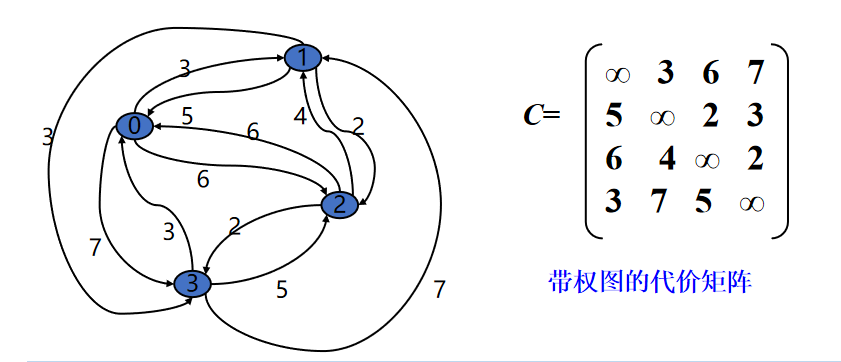2、【应用】
例如：校车怎样以最短的路线行走而接送到所有学生？报纸和牛奶的配送路线怎样最优？循环旅游怎样选取才能实现开支最少？公司视察子公司怎样出差更高效？
3、【蛮力法求解】
用蛮力法解决TSP问题，可以找出所有可能的旅行路线，即依次考察图中所有顶点的全排列，从中选取路径长度最短的简单回路。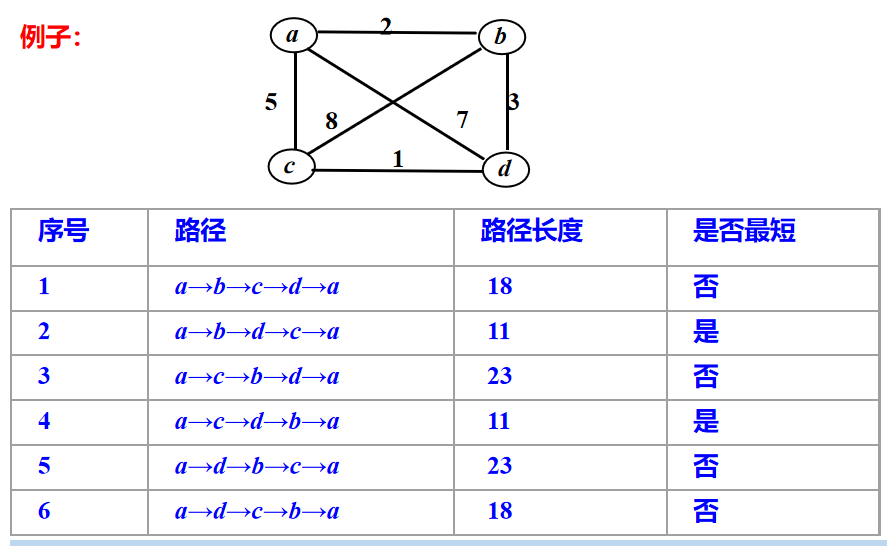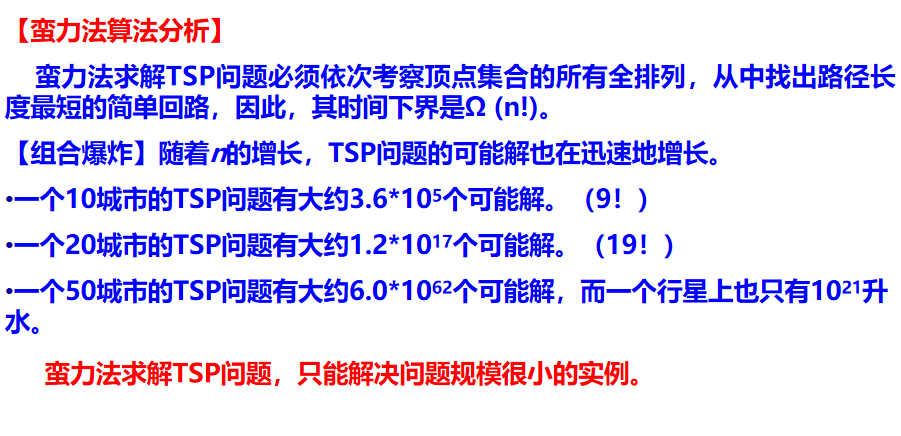4、证明TSP问题满足最优性原理
设s,s1,s2, …,sp,s是从s出发的一条路径长度最短的简单回路，假设从s到下一个城市s1已经求出，则问题转化为求从s1到s的最短路径，显然s1,s2,…,sp,s一定构成一条从s1到s的最短路径。
如若不然，设s1,r1,r2,…,rq,s是一条从s1到s的最短路径且经过n-1个不同城市，则s,s1,r1,r2,…,rq,s将是一条从s出发的路径长度最短的简单回路且比s,s1,s2,…,sp,s要短，从而导致矛盾。所以，TSP问题满足最优性原理。
5、动态规划法求解过程——示例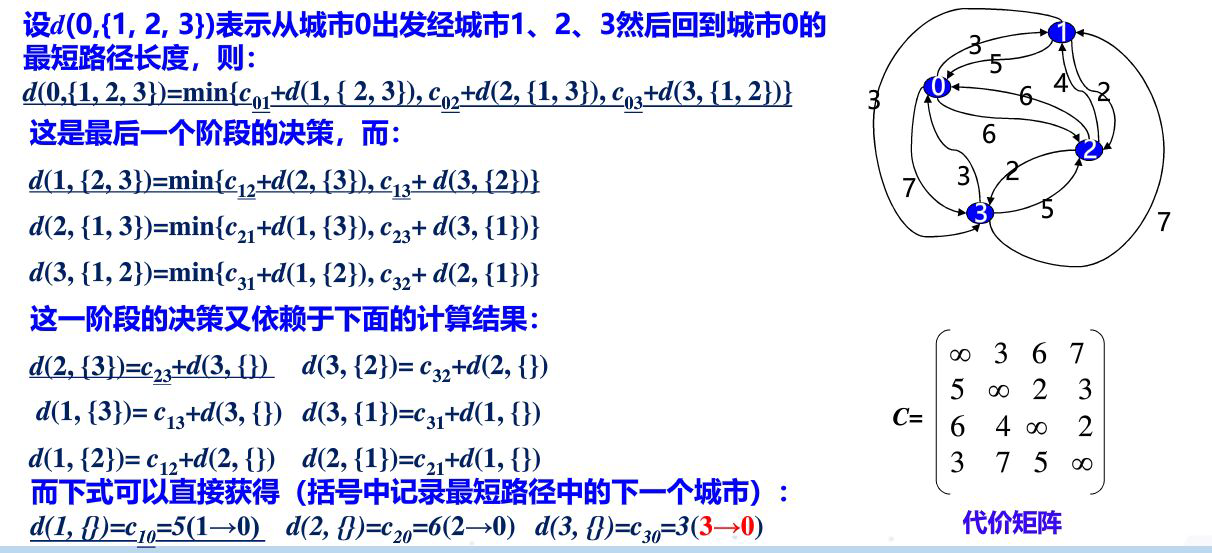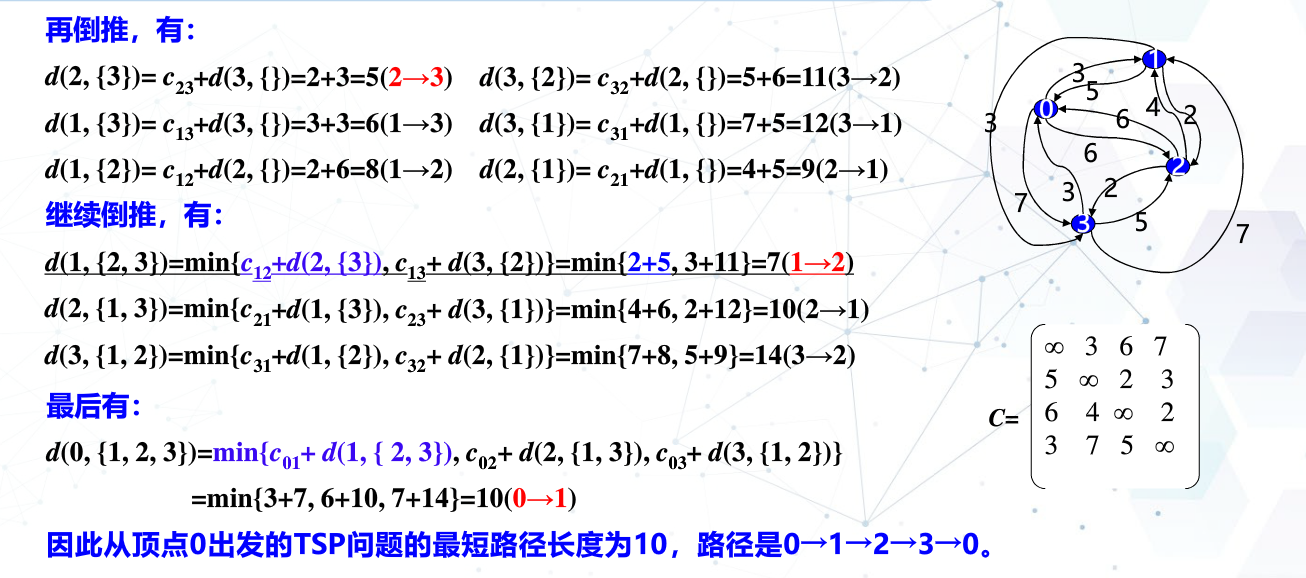6、动态规划求解问题的步骤
（1）划分子问题—找到“状态”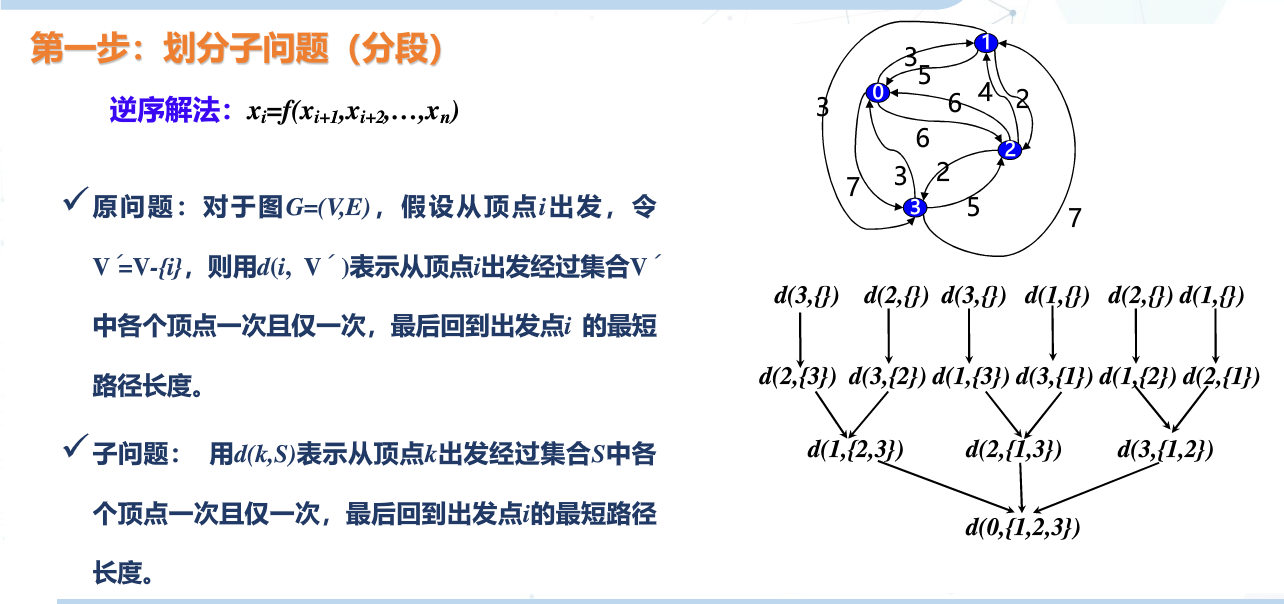（2）状态方程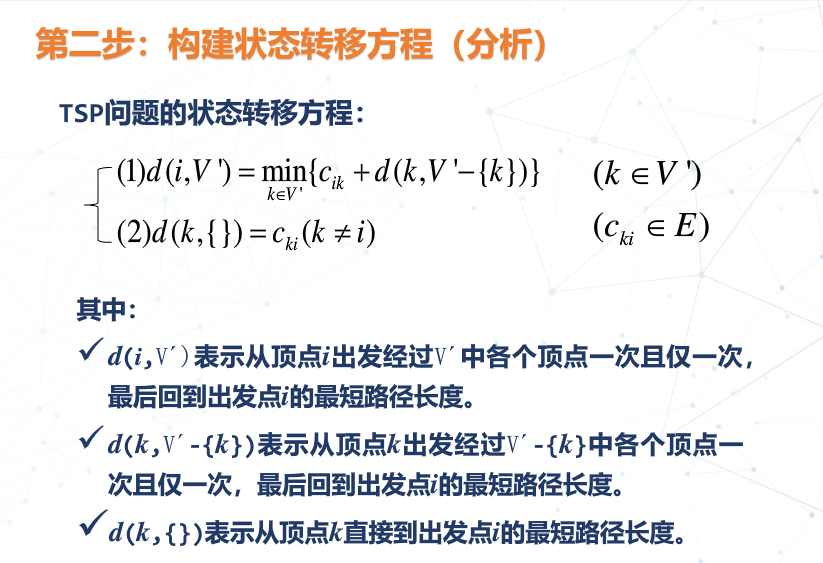（3）填表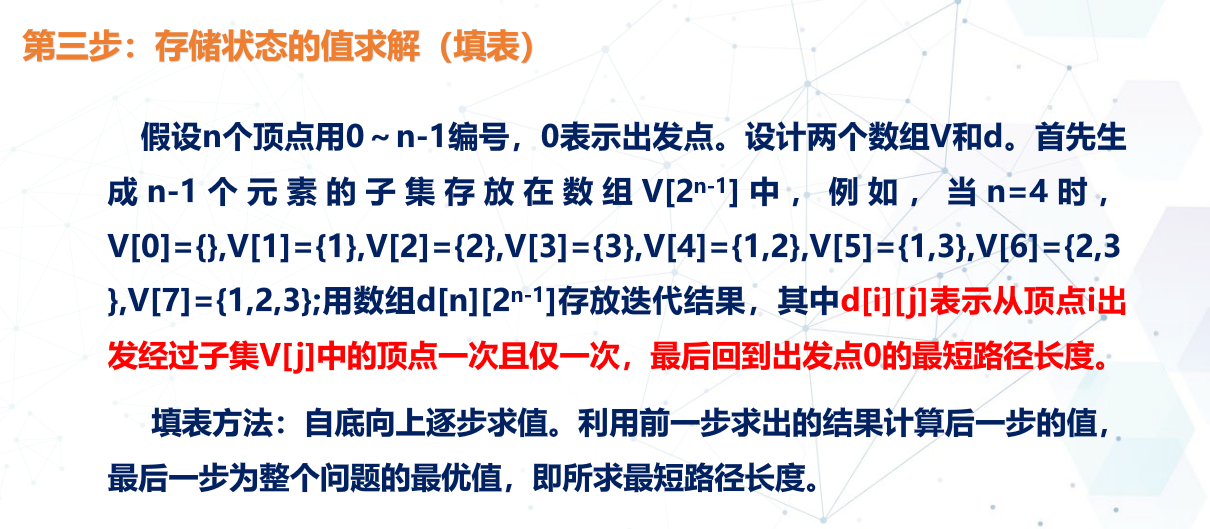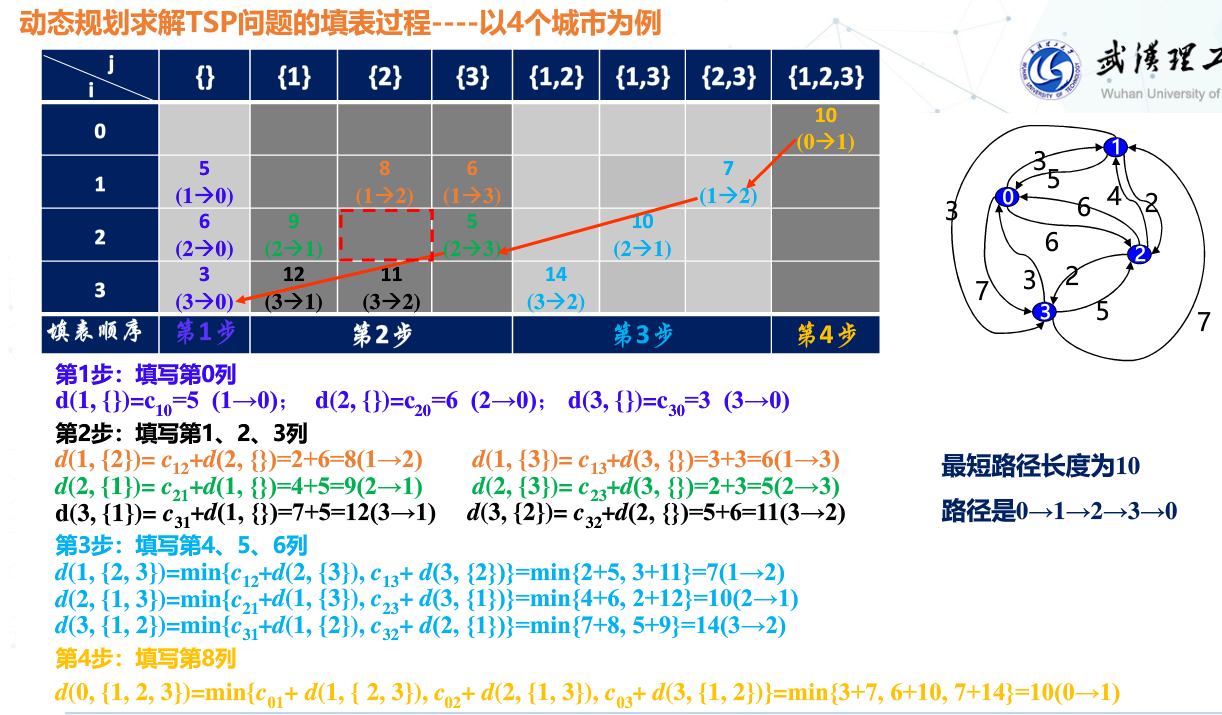7、算法设计
（1）【数据结构设计】
二维数组c[n][n]存放顶点之间的代价，n个顶点用0～n-1的数字编号
一维数组V[2n-1]存放1～n-1个元素的所有子集
二维数组dp[n][2n-1]存放递推结果，其中dp[i][j]表示从顶点i经过子集V[j]中的顶点一次且仅一次，最后回到出发点0的最短路径长度。
（2）【动态规划法算法】
算法——TSP问题
1．for(i=1; i<n; i++) d[i]=c[i]; //初始化第0列
2．for(j=1; j<2n-1-1; j++)
for(i=1; i<n; i++) //依次进行第i次迭代
if(子集V[j]中不包含i)
对V[j]中的每个元素k，计算d[i][j]=min(c[i][k]+d[k][j-1]);
3.对V[2n-1-1]中的每一个元素k，计算d[2n-1-1]=min(c[k]+d[k][2n-1-2]);
4．输出最短路径长度d[2n-1-1];

8、算法分析展开全文• 这篇文章的代码是笔者自己用动态规划的思想用matlab实现的，里面的用到了矩阵运算和matlab内置函数的使用，相比c写起来代码少了很多，数学好的看起来应该更加简单易懂。 但是是根据一位大牛的文章写的，这里附上他...matlab
• 解题思路主要有两部分：第一部分：i为当前节点(城市)，S为还没有遍历的节点(城市集合)，表示从第i个节点起，经历S集合中所有的点，到达终点的最短路径长度。因此有：第二部分，回溯，找到...代码如下：# tsp问题clas...
• 本压缩文档包含三个文件：用动态规划法解决TSP问题可执行源代码，word文档报告，实验测试数据
• ## 【动态规划法】求解TSP问题

万次阅读 多人点赞 2019-07-14 23:50:18
假设从顶点i出发，令d(i, V’ )表示从顶点i出发经过V’ 中各个顶点一次且仅一次，最后回到出发点（i）的最短路径长度，开始时， V’ ＝V－{i}，于是，TSP问题动态规划函数为： d(i, V’ )=min{cik+d(k, V’ －{k})...
• 动态规划的方法的最大难点就在于初始变量的确定，选择合适的初始变量才能更好的运用动态规划的方式解决问题。我在这里定义的变量就是d(i,S),设s出发点，其中i是一个点,而S是点的集合，这个变量的意思就是从i出发，...
• 某推销员要从城市v1出发，访问其它城市v2，v3，…，v6各一次且仅一次，最后返回v1。D为各城市间的距离矩阵。 问：该推销员应如何选择路线，才能使总的行程最短？
• 【TSP问题】基于hopfield神经网络求解TSP问题matlab.md
• 一、Tsp问题 假设有一个旅行商人要拜访n个城市，他必须...二、动态规划 设s, s1, s2, …, sp, s是从s出发的一条路径长度最短的简单回路，假设从s到下一个城市s1已经求出，则问题转化为求从s1到s的最短路径，显然...
• 经典算法问题-TSP商旅问题（Traveling Salesman Problem），它是数学领域中著名问题之一。...代码包含遗传算法和动态规划求解这个问题，里面有完整源代码，并且有详细注释，还有两者的比较分析。
• 此文章借鉴于博文...一、问题 二、想法 三、讲解 1、怎么求顶点子集，即这些怎么记录？答：例如4个顶点{0,1,2,3}，{1,2,3}依次为{}，{1}，{2}，{1,2}，{3}，{1,3}，{2,3}，{1,2,3}。十进制数0、...c++ 算法
• C++ 动态规划求解TSP（旅行商问题） 介绍了动态规划求解问题的四个步骤，按步骤分析求解TSP，给出了使用C++求解TSP问题的源代码数学 算法 c++
• TSP问题是指假设有一个旅行商人要拜访n个城市，他必须选择所要走的路径，路径的限制是每个城市只能拜访一次，而且最后要回到原来出发的城市。路径的选择目标是要求得的...本文使用蚁群算法求解TSP问题（matlab代码）
• 首先，什么是TSP问题？直接摘百度—— 旅行商问题，即TSP问题(Traveling Salesman Problem)又译为旅行推销员问题、货郎担问题，是数学领域中著名问题之一。假设有一个旅行商人要拜访n个城市，他必须选择所要走的...
• MATLAB源码集锦-蚁群算法求解TSP问题matlab代码
• TSP问题是指假设有一个旅行商人要拜访n个城市，他必须选择所要走的路径，路径的限制是每个城市只能拜访一次，而且最后要回到原来出发的城市。路径的选择目标是要求得的路径路程为所有路径之中的最小值。本文使用遗传...
• 采用C-TSP 问题和TSPLIB 数据库中的多组TSP 问题作为实验用算例, 并将所提出算法与其他5 种智能优化算法进行对比, 仿真结果表明, 所提出算法在求解准确率、稳定性和所需迭代次数等方面具有相对优势.</p>
• distence_matrix = np.zeros([n,n]) for i in range(0, n): for j in range(n): distence = distance(cities[i],cities[j]) distence_matrix[i][j] = distence 使用动态规划求解TSP问题： S = Solution(distence_...
• 本介绍用python解决TSP问题的第二个方法——动态规划法算法介绍动态规划算法根据的原理是，可以将原问题细分为规模更小的子...我使用DP求解TSP问题的主要分为三个主要部分：1)假定我们从城市0出发，经过了所有城市...
• 用两种方法通过python编程对TSP问题求解 , 一是通过gurobi求解求解 , 二是通过智能算法PSO(粒子群算法)进行求解 . 并画出最优路径 . 资源中包括TSP问题的数学模型 , 两种求解方法的python代码 , 以及求解结果图...
• 基于连续型Hopfield神经网络求解TSP问题 matlab实现 适合初学者学习研究
• TSP问题是指旅行家要旅行n个城市，要求各个城市经历且仅经历一次，然后回到出发城市，并要求所走路程最短。 解决思路： 以四个城市为例讲解 假设n个顶点用0~ n-1个数字编号，首先要生成1~ n-1个元素的子集存放在...
• 动态规划TSP问题（状态压缩dp）TSP问题简述  给定图上若干个点，以及他们之间的距离，求一条距离和最小的回路，使得该回路正好经过每个点一次。TSP也叫旅行商问题、货郎担问题。。。状态转移方程  用 V’ 表示...状态压缩...